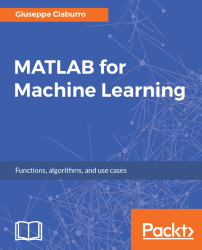•#### MATLAB for Machine Learning#### Overview of this book

MATLAB is the language of choice for many researchers and mathematics experts for machine learning. This book will help you build a foundation in machine learning using MATLAB for beginners. You’ll start by getting your system ready with t he MATLAB environment for machine learning and you’ll see how to easily interact with the Matlab workspace. We’ll then move on to data cleansing, mining and analyzing various data types in machine learning and you’ll see how to display data values on a plot. Next, you’ll get to know about the different types of regression techniques and how to apply them to your data using the MATLAB functions. You’ll understand the basic concepts of neural networks and perform data fitting, pattern recognition, and clustering analysis. Finally, you’ll explore feature selection and extraction techniques for dimensionality reduction for performance improvement. At the end of the book, you will learn to put it all together into real-world cases covering major machine learning algorithms and be comfortable in performing machine learning with MATLAB.
Title PageCreditsForewordwww.PacktPub.comCustomer FeedbackPrefaceFree Chapter
Getting Started with MATLAB Machine LearningImporting and Organizing Data in MATLABFrom Data to Knowledge DiscoveryFinding Relationships between Variables - Regression TechniquesPattern Recognition through Classification AlgorithmsIdentifying Groups of Data Using Clustering MethodsSimulation of Human Thinking - Artificial Neural NetworksImproving the Performance of the Machine Learning Model - Dimensionality ReductionMachine Learning in Practice## Chapter 2. Importing and Organizing Data in MATLAB

Today, the amount of data generated is enormous; smartphones, credit cards, televisions, computers, home appliances, sensors, domotic systems, public and private transport, and so on are just a few examples of devices that generate data seamlessly. Such data is stored and then used for various purposes. One of these is data analysis using machine learning algorithms.

In the previous chapter, we analyzed how to build machine learning models step by step. At the start of the workflow, there is organization of data. Indeed, after collecting data, we typically need to import and preprocess. This first step is crucial for the proper functioning of the model that we will build and then for the final result.

In this chapter, we will have a look at how to import and organize our data in MATLAB. To do this, you should familiarize yourself with the MATLAB workspace in order to make the operations as simple as possible. Then we will analyze the different...